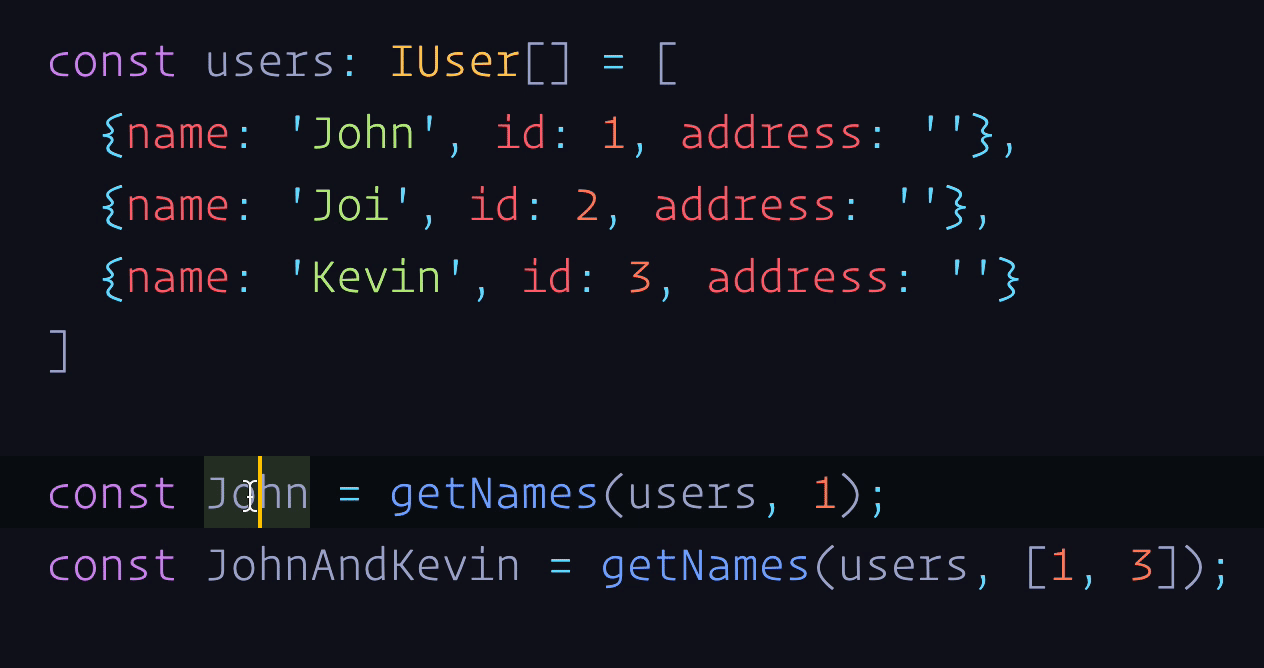`C++` `Java` 这些静态语言中，可以通过为一个函数定义多个不同的签名，也即不同输入和输出来达到函数的重载。但是 `JavaScript` 的函数是没有签名的，它的输入参数是由包含零个或者多个值的数组来表示的，并且 JS 不定义参数的类型，也不检查接收的参数的类型和个数，所以在 JavaScript 中是不可能实现真正的函数重载的。

`TypeScript` 作为 `JavaScript` 的超集，既允许我们对函数参数的类型进行定义，也可以进行函数重载。那么在什么情况我们会需要函数的重载呢？假设有一个这样的场景，我们需要一个函数，它接收一个用户列表做为第一个参数，用户 `id` 作为第二个参数，过滤出`id`所对应的用户名，如果`id`是一个数字，就返回该用户名字字符串，如果`id`是一个列表，则返回一个用户姓名列表，很容易得到以下定义：

``````interface IUser {
name: string;
id: number;
// ...
}

const getNames = (userList: IUser[], id: number | number[]): string | string[] => {
if (Array.isArray(id)) {
const names: string[] = [];
userList.forEach((user: IUser) => {
if (id.includes(user.id)) {
names.push(user.name);
}
})
return names;
}
const found = userList.find((user: IUser) => user.id === id);
if (found) {
return found.name;
}
return ''
}
``````

``````const users: IUser[] = [
{name: 'John', id: 1, address: ''},
{name: 'Joi', id: 2, address: ''},
{name: 'Kevin', id: 3, address: ''}
]
const John = getNames(users, 1);
const JohnAndKevin = getNames(users, [1, 3]);
````````````function getNames(userList: IUser[], id: number): string;
function getNames(userList: IUser[], id: number[]): string[];
function getNames(userList: IUser[], id: number | number[]): string | string[]{
if (Array.isArray(id)) {
const names: string[] = [];
userList.forEach((user: IUser) => {
if (id.includes(user.id)) {
names.push(user.name);
}
})
return names;
}
const found = userList.find((user: IUser) => user.id === id);
if (found) {
return found.name;
} else {
return ''
}
}
``````

## 重载的顺序

``````interface ReadonlyArray<T> {
reduce(callbackfn: (previousValue: T, currentValue: T, currentIndex: number, array: readonly T[]) => T): T;
reduce(callbackfn: (previousValue: T, currentValue: T, currentIndex: number, array: readonly T[]) => T, initialValue: T): T;
reduce<U>(callbackfn: (previousValue: U, currentValue: T, currentIndex: number, array: readonly T[]) => U, initialValue: U): U;
}
``````

``````const A = [1, '2', 3]
const str: string = A.reduce((str, a) => `\${str} \${a.toString()}`, '')
// const str: string
// Type 'string | number' is not assignable to type 'string'.
// Type 'number' is not assignable to type 'string'
``````

``````const A = [1, '2', 3]
const str: string = A.reduce<string>((str, a) => `\${str} \${a.toString()}`, '')
``````